#### Infinite grid sequences

Now suppose that the number,, of samples is not given. The task is to define an infinite sequence that has the nice properties of the van der Corput sequence but works for any dimension. This will become the notion of a multi-resolution grid. The resolution can be iteratively doubled. For a multi-resolution standard grid in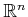, the sequence will first place one point at the origin. After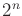points have been placed, there will be a grid with two points per axis. After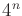points, there will be four points per axis. Thus, after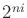points for any positive integer, a grid with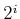points per axis will be represented. If only complete grids are allowed, then it becomes clear why they appear inappropriate for high-dimensional problems. For example, if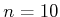, then full grids appear after,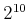,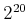,, and so on, samples. Each doubling in resolution multiplies the number of points by. Thus, to use grids in high dimensions, one must be willing to accept partial grids and define an infinite sequence that places points in a nice way.

The van der Corput sequence can be extended in a straightforward way as follows. Suppose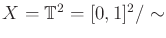. The original van der Corput sequence started by counting in binary. The least significant bit was used to select which half of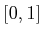was sampled. In the current setting, the two least significant bits can be used to select the quadrant of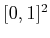. The next two bits can be used to select the quadrant within the quadrant. This procedure continues recursively to obtain a complete grid after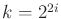points, for any positive integer. For any, however, there is only a partial grid. The points are distributed with optimal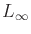dispersion. This same idea can be applied in dimensionby usingbits at a time from the binary sequence to select the orthant (-dimensional quadrant). Many other orderings produce-optimal dispersion. Selecting orderings that additionally optimize other criteria, such as discrepancy or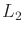dispersion, are covered in [639,644]. Unfortunately, it is more difficult to make a multi-resolution Sukharev grid. The base becomes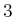instead of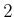; after every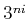points a complete grid is obtained. For example, in one dimension, the first point appears at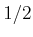. The next two points appear at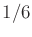and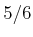. The next complete one-dimensional grid appears after there are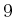points.

Steven M LaValle 2020-08-14